### Showing videos fromEddie Woo with a total of 3,931 videos

05:01Using the Sine Rule to Find Angles (3 of 3: Searching for ambiguity)
3y5m ago
06:26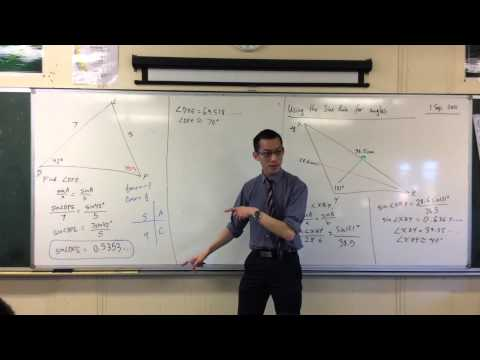Using the Sine Rule to Find Angles (2 of 3: The Ambiguous Case)
3y5m ago
05:12Using the Sine Rule to Find Angles (1 of 3: Basic Example)
3y5m ago
05:54Solving Trigonometric Equations by Quadrants (3 of 3: Why 180°+ x?)
3y6m ago
07:46Solving Trigonometric Equations by Quadrants (2 of 3: Examples)
3y6m ago
04:42Solving Trigonometric Equations by Quadrants (1 of 3: Introduction)
3y6m ago
05:36Solving Trigonometric Equations by Graphs (2 of 2: Tan example)
3y6m ago
04:01Solving Trigonometric Equations by Graphs (1 of 2: Cosine example)
3y6m ago
09:47Parametric Equation of Chord (2 of 2: Point-Gradient Form)
3y6m ago
13:02Thinking Deeply About Factors (3 of 3: Finding Explanations)
3y6m ago
10:07Thinking Deeply About Factors (1 of 3: Initial Observations)
3y6m ago
10:17Solving Trigonometric Equations (2 of 2: Finding the Solutions)
3y6m ago
09:15Solving Trigonometric Equations (1 of 2: Thinking Graphically)
3y6m ago
08:39Introduction to Parametrics (2 of 3: What Parameters Actually Are)
3y6m ago
09:19Introduction to Parametrics (1 of 3: Why Parameters Are Useful)
3y6m ago
12:08Evaluating Specific Binomial Coefficients (Exam Questions)
3y6m ago
07:25The Travelling Salesman (3 of 3: Ant Colonisation Heuristic)
3y6m ago
11:23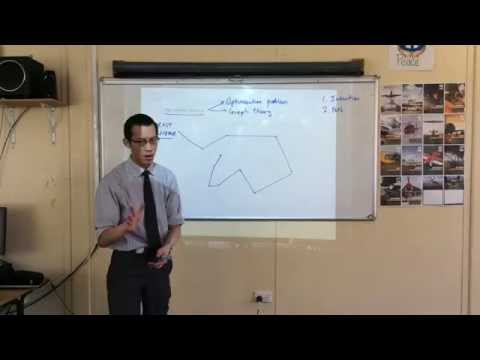The Travelling Salesman (2 of 3: Nearest Neighbour & SFCs)
3y6m ago
07:07The Travelling Salesman (1 of 3: Understanding the Problem)
3y6m ago
09:36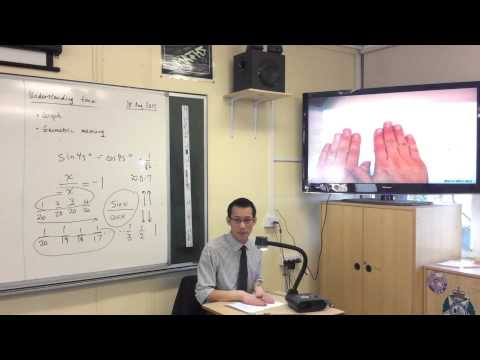Understanding the graph of tan(x) (2 of 2: Geometric Features)
3y6m ago
12:26Understanding the graph of tan(x) (1 of 2: Thinking Visually)
3y6m ago
06:23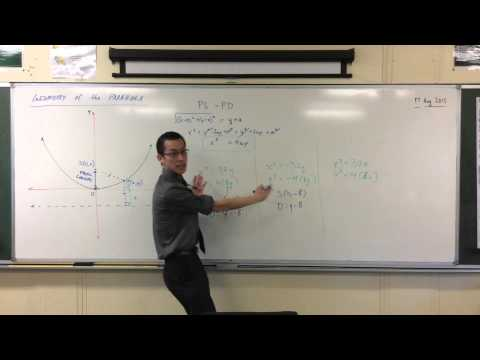Standard Form of a Parabola (2 of 3: Determining the Focus & Directrix)
3y6m ago
08:34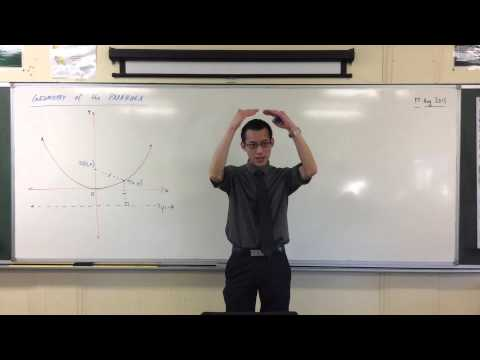Standard Form of a Parabola (1 of 3: From the Locus Definition)
3y6m ago
06:48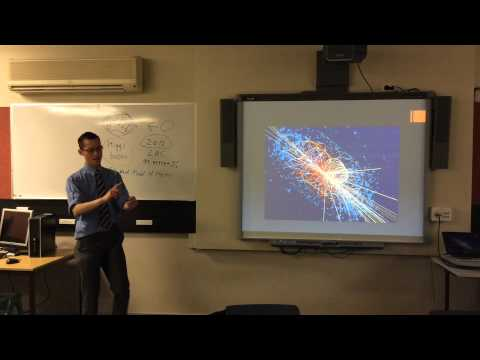Why is there mathematics in STEM? (3 of 3: The Higgs Boson)
3y6m ago
12:30Why is there mathematics in STEM? (1 of 3: Different Kinds of Knowledge)
3y6m ago